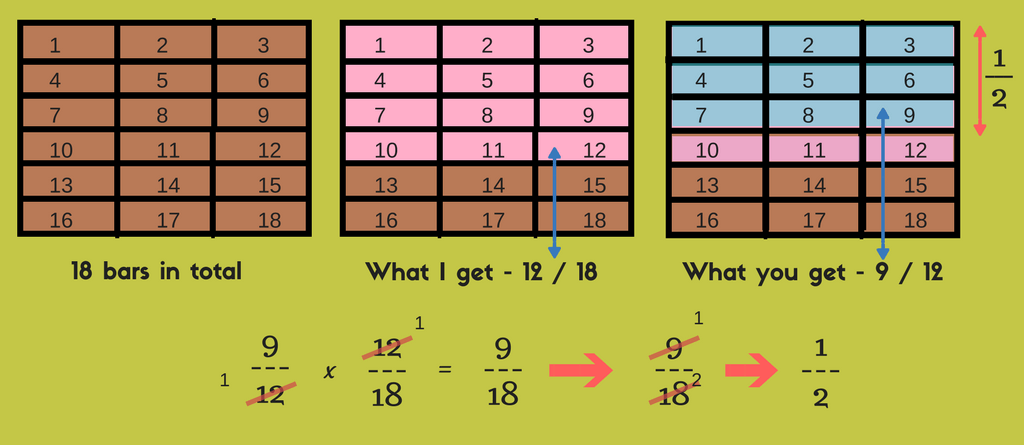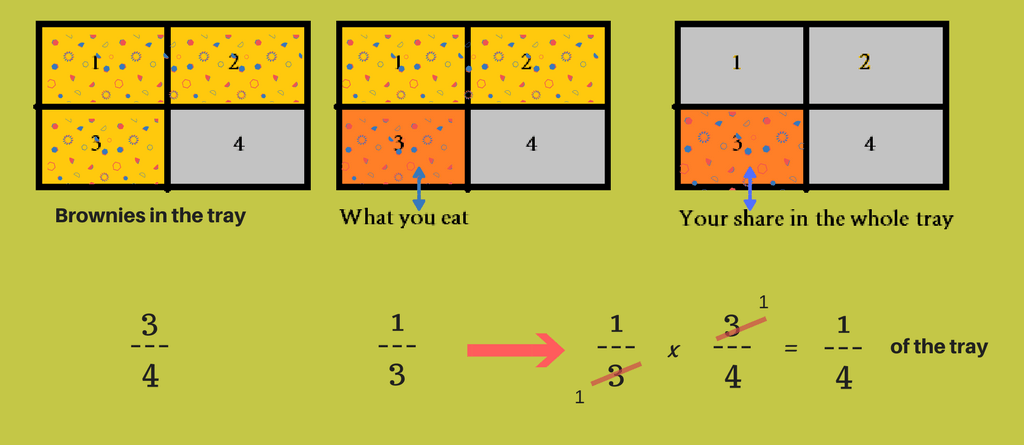# How to multiply fractionsLearn multiplying fractions in simple steps. Scroll through the examples and know how to multiply fractions. What’s more? Well, we have FREE math resources and more to help you in practicing multiplying fractions. So, let’s get started.

## Multiplying fractions when the numerator of one equals or is a factor of the denominator of the other

Let’s take an example –

Problem –  My mother bought 18 bars of yummy chocolate. Of which, my mother gave me 12/18 of the bar. Now, I know you like chocolates too. And so, I gave you 9/12 of what I have (12/18). How much did you get in total?

Solution –

### Step 1

Can you see that 12 is in the denominator of one fraction and the numerator of the other?Good, now divide them with each other.

Resulting Fraction =

### Step 2

Good work! Now look at the present fraction, here 18 is divisible by 9 (9 x 2 = 18).

Resulting fraction =

### Step 3

Check to see if the resulting fraction could be further simplified or not.1/2 is in it’s simplest fraction form.This was the step by step solution for multiplying fractions, hope it helped and made your calculations easy.

Let’s try another one!

Problem – 3/4 of a tray of brownies was sitting on the counter. You decided to eat 1/3 of the brownies in the tray. How much of the whole tray of brownies did you eat?

Solution –

### Step 1

Can you see that 3 is in the denominator of one fraction and the numerator of the other?Good, now divide them with each other.

Related text  How to get wet

Resulting Fraction =

### Step 2 & 3

Good work! Now look at the present fraction, it can’t be simplified further more.

It is in it’s simplest fraction form.## Multiplying Fractions – More Resources

### Free Online Fraction Calculator

Well, fraction multiplication is actually too easy and quick. What’s quicker is the calculation of fractions in fraction calculator. The answer is provided with steps for better understanding. Try Fraction calculator now.

### Free Math Worksheets

Fraction worksheets can give you fun practice experience. These math worksheets have story problems that helps you to relate to and understand the problem. Download fraction worksheets for free.

### Math Games

There is nothing better than play based learning. Make fraction practice fun and interesting while you play with Froggy Fraction and Monkey Fraction. It helps in learning, understanding, practicing, bonding and having fun while you play. (Also available on Amazon).

So, here’s hoping that this blog helped you with understanding multiplying fractions. If any doubts, do share with us. Do use math resources to get better practice in a fun way.  If you are confused with more fraction problems with different mathematical operation, try ‘understanding adding and subtracting fractions’ and ‘dividing fractions‘. See you.

Understanding Multiplying Fractions With ExamplesJune 23, 2017Understanding Multiplying Fractions With ExamplesJune 23, 2017